Neha J.

Jamia Nagar, Delhi, India - 110025# Neha J.

## Maths teacher

Jamia Nagar, Delhi, India - 110025.

4.9

UrbanPro Rating

Details verified of Neha J.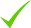IdentityEducation

Know how UrbanPro verifies Tutor details

Identity is verified based on matching the details uploaded by the Tutor with government databases.

## Overview

I have 2 years coaching experience. I have taught 9th to 12th & B.sc mathematics also.

English

Hindi

## Education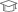Mjpru 2015

Master of Science (M.Sc.)Mjpru 2017

Bachelor of Education (B.Ed.)

Jamia Nagar, Delhi, India - 110025

## Verified Info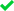ID VerifiedPhone VerifiedEmail Verified

## Demo Class

No

Report this Profile

Is this listing inaccurate or duplicate? Any other problem?

Please tell us about the problem and we will fix it.

Please describe the problem that you see in this page.

Type the letters as shown below *

Please enter the letters as show below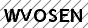## Class 11 Tuition Overview

Class 11 Tuition

Class Location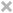Student's HomeTutor's HomeOnline (video chat via skype, google hangout etc)

Years of Experience in Class 11 Tuition

2

Board

CBSE, State

CBSE Subjects taught

Mathematics

Taught in School or College

Yes

State Syllabus Subjects taught

Mathematics

Teaching Experience in detail in Class 11 Tuition

o

## Reviews

this is test message this is test message this is test message this is test message this is test message this is test message this is test message

No Reviews yet! Be the first one to Review

## FAQs

1. Which classes do you teach?

I teach Class 10 Tuition, Class 11 Tuition, Class 12 Tuition, Class 8 Tuition and Class 9 Tuition Classes.

2. Do you provide a demo class?

No, I don't provide a demo class.

3. How many years of experience do you have?

I have been teaching for 2 years.

## Answers by Neha J. (1)

Answered on 25 Jun

We have the formula x^3+y^3+z^3-3xyz=(x+y+z)(x^2+y^2+z^2-xy-yz-zx) From question-x^2+y^2+z^2=xy+yz+zx Putting the value in formula- x^3+y^3+z^3-3xyz=(x+y+z)(xy+yz+zx-xy-yz-zx) =>x^3+y^3+z^3-3xyz=(x+y+z)(0) =>x^3+y^3+z^3-3xyz=0 =>x^3+y^3+z^3=3xyz Ans. ...more

We have the formula

x^3+y^3+z^3-3xyz=(x+y+z)(x^2+y^2+z^2-xy-yz-zx)

From question-x^2+y^2+z^2=xy+yz+zx

Putting the value in formula-

x^3+y^3+z^3-3xyz=(x+y+z)(xy+yz+zx-xy-yz-zx)

=>x^3+y^3+z^3-3xyz=(x+y+z)(0)

=>x^3+y^3+z^3-3xyz=0

=>x^3+y^3+z^3=3xyz Ans.

Dislike Bookmark

Neha J.Directions

x
Class 11 Tuition 4.9

Class LocationStudent's HomeTutor's HomeOnline (video chat via skype, google hangout etc)

Years of Experience in Class 11 Tuition

2

Board

CBSE, State

CBSE Subjects taught

Mathematics

Taught in School or College

Yes

State Syllabus Subjects taught

Mathematics

Teaching Experience in detail in Class 11 Tuition

o

Class 12 Tuition 4.9

Class LocationStudent's HomeTutor's HomeOnline (video chat via skype, google hangout etc)

Years of Experience in Class 12 Tuition

2

Board

CBSE, State

CBSE Subjects taught

Mathematics

Taught in School or College

Yes

State Syllabus Subjects taught

Mathematics

Class 10 Tuition 4.9

Class LocationStudent's HomeTutor's HomeOnline (video chat via skype, google hangout etc)

Years of Experience in Class 10 Tuition

2

Board

CBSE, State

CBSE Subjects taught

Mathematics

Taught in School or College

Yes

State Syllabus Subjects taught

Mathematics

Class 9 Tuition 4.9

Class LocationStudent's HomeTutor's HomeOnline (video chat via skype, google hangout etc)

Years of Experience in Class 9 Tuition

2

Board

CBSE, State

CBSE Subjects taught

Mathematics

Taught in School or College

Yes

State Syllabus Subjects taught

Mathematics

Teaching Experience in detail in Class 9 Tuition

o

Class 8 Tuition 4.9

Class LocationStudent's HomeTutor's HomeOnline (video chat via skype, google hangout etc)

Years of Experience in Class 8 Tuition

2

Board

State, CBSE

CBSE Subjects taught

Mathematics

Taught in School or College

Yes

State Syllabus Subjects taught

Mathematics, Science

this is test message this is test message this is test message this is test message this is test message this is test message this is test message

No Reviews yet! Be the first one to Review

## Answers by Neha J. (1)

Answered on 25 Jun

We have the formula x^3+y^3+z^3-3xyz=(x+y+z)(x^2+y^2+z^2-xy-yz-zx) From question-x^2+y^2+z^2=xy+yz+zx Putting the value in formula- x^3+y^3+z^3-3xyz=(x+y+z)(xy+yz+zx-xy-yz-zx) =>x^3+y^3+z^3-3xyz=(x+y+z)(0) =>x^3+y^3+z^3-3xyz=0 =>x^3+y^3+z^3=3xyz Ans. ...more

We have the formula

x^3+y^3+z^3-3xyz=(x+y+z)(x^2+y^2+z^2-xy-yz-zx)

From question-x^2+y^2+z^2=xy+yz+zx

Putting the value in formula-

x^3+y^3+z^3-3xyz=(x+y+z)(xy+yz+zx-xy-yz-zx)

=>x^3+y^3+z^3-3xyz=(x+y+z)(0)

=>x^3+y^3+z^3-3xyz=0

=>x^3+y^3+z^3=3xyz Ans.

Dislike Bookmark

Neha J. describes herself as Maths teacher. She conducts classes in Class 10 Tuition, Class 11 Tuition and Class 12 Tuition. Neha is located in Jamia Nagar, Delhi. Neha takes Regular Classes- at her Home and Online Classes- via online medium. She has 2 years of teaching experience . Neha has completed Master of Science (M.Sc.) from Mjpru in 2015 and Bachelor of Education (B.Ed.) from Mjpru in 2017. She is well versed in English and Hindi.

Recommended Profiles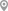Jamia Nagar, DelhiJamia Nagar, DelhiJamia Nagar, DelhiJamia Nagar, DelhiJamia Nagar, DelhiJamia Nagar, Delhi

X

Post your Learning Need

Let us shortlist and give the best tutors and institutes.

or

Send Enquiry to Neha J.

Let Neha J. know you are interested in their class

Reply to 's review